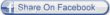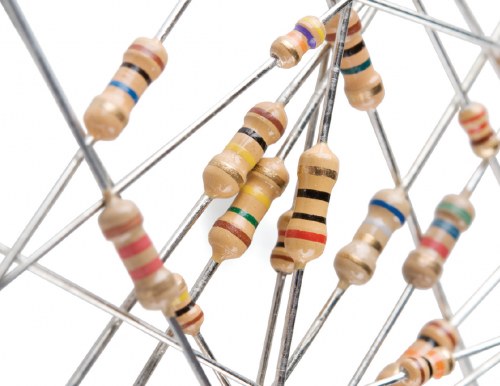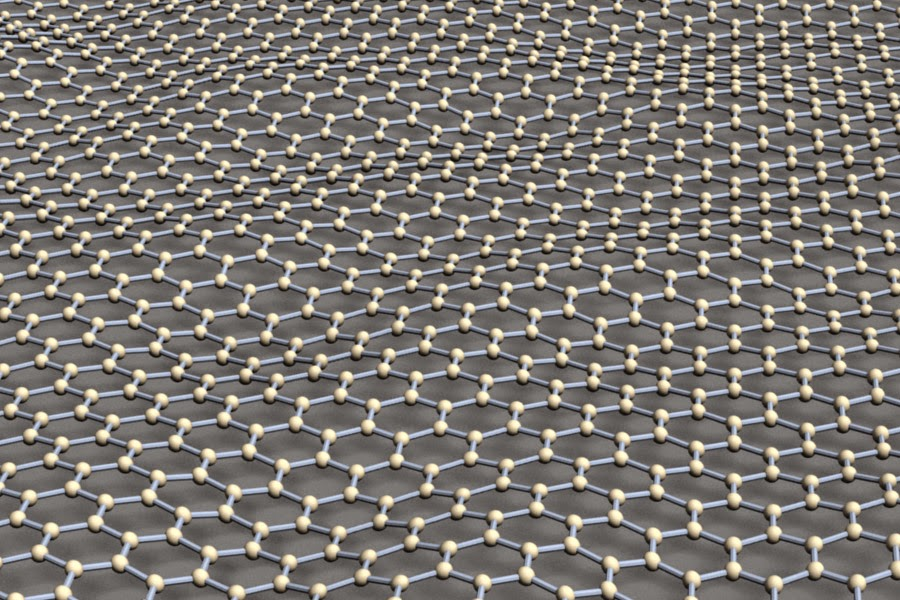## Friday, 23 January 2015

### Electrical Conductivity and ResistivityI guess “conductivity” and “resistivity” are terms relatively simple to explain to the general public. Conductive materials conduct electrical currents easily, and resistive materials resist electrical currents. So what should an engineer know about these terms that the general public does not generally know? By knowing how they are defined, and knowing the difference between resistivity and conductivity, and resistance and conductance.No, this is not about "Resistance: Fall of Man" game (image taken from Best Game Wallpapers).

Definition

Terminology: Electrical Resistivity; ResistivitySpecific Electrical ResistanceVolume Resistivity
Definition:      a material’s intrinsic ability to oppose electrical current flowing through it
Symbol:          ρ
S.I. Unit:         ohm⋅meter (Ω⋅m)

Terminology: Electrical Conductivity; Specific Conductance
Definition:     a material’s intrinsic ability to conduct electrical current through it
Symbol:         σ (occasionally κ and γ)
S.I. Unit:        siemens per meter (S/m)The universal understanding about resistance in an image... (image taken from Sparkfun).

Most resistors and conductors are made of a single material (instead of a composite) and have uniform cross section with a uniform flow of electrical current (think copper wires). The electrical resistivity is defined as:R = electrical resistance of a uniform cross section material; unit is ohm (Ω)
A = cross section area of the material; unit is square meters (m2)
l = length of material; unit is meters (m)

Resistance is then found through:
Conductivity is calculated as the inverse of resistivity:As an engineer, it is imperative to understand that “resistance” and “resistivity” are different terms. Resistivity is an intrinsic material property, just like Young’s modulus, and is independent on the material geometry. “Resistance” however is a geometrical property. The resistivity of a pure copper wire is always constant (1.68×10−8 Ω⋅m) because this is pure copper’s property, but the wire’s resistance between both ends is higher when the wire gets longer. The same understanding should be applied to both “conductance” and “conductivity”.3D rendering of a graphene sheet, a material with the lowest resistivity to date (image taken from James Hedberg).

Interesting facts

As engineers, we should be aware of some material facts related to resistivity less we become laughing stocks. Here are some interesting facts that some engineers may miss, especially if their job need not deal too much with this property.

• Resistivity of a material is temperature dependant, so generally the value is listed in room temperature of 20°C. An increase in temperature will generally see the resistivity increase in metals and decrease in semiconductors.
• Among the 3 precious metals most used in electrical conductors, Silver (1.59×10−8 Ω⋅m) has the lowest resistivity compared to Copper (1.68×10−8 Ω⋅m) and Gold (2.44×10−8 Ω⋅m). The reason it is used less extensively is because Copper is cheaper and Gold is more corrosion resistant.
• Semiconductors and electrolytes (solution of water) have variable resistivity; the former is dependent on a large variety of factors while the latter is dependent on the concentration of dissolved salts.
• Superconductors have the ideal resistivity of 0.
• Carbon in the form of graphite has lower resistivity (2.5 – 5.0×10−6 Ω⋅m parallel to basal plane) than in diamond form (1×1012 Ω⋅m), showing how the same element could have different resistivity in different form. However diamond is still more conductive than air (1.30 – 3.30×1016 Ω⋅m).
• The material with the lowest resistivity in room temperature is graphene (1.00×10−8 Ω⋅m), which is pure carbon in sheet form as thick as an atom.A piece of magnet levitating above a superconductor. Superconductors work at very low temperatures and have a resistivity of 0 (image taken from New Scientists).

A very useful comparison of the resistivity and conductivity of most materials could be viewed from Wikipedia (where else).

Sources:

#### 1 comment:

1.thansk very good post
gear reduction box
http://alemdaroglumakina.com/en/